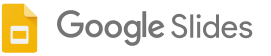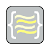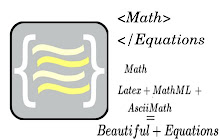Check out the store for many more# Math Equations

(268)
98,406 users

## OverviewConvert Latex, AsciiMath and MathML equations to an image.
```Math Equations allows you to take your typeset languages and convert them to images to use inside of your slideshow.  Other features include the ability to reload a equation image and make changes and re upload to your presentation.

Recent changes added the math equations data into the alt text, which you can see by clicking using control + alt + y.

Supported Languages are
-Latex
-AsciiMath
-MathML

Other Features
-Font Color
-Font Size

If you have any problems just send my a message at
bafeaturerequest@gmail.com
Code can be located at

If this extension is interested you can check out my other extension that allows you to create math equations on any site.

Examples
Tex
--valid Tex equation

x^n + y^n = z^n

Tex type uses inline notation.  Which restricts what you can add in your equation.

Add \[ ]\ will cause a error at the current iteration.
Also many formatting tags and the use of usepackage will cause a error. Some of these feature i will include after i build a separate window to work on larger equations.

MathML
--Valid MathML

<mi>&#x3C0;</mi>
<mo>&#x2062;</mo>
<msup>
<mi>r</mi>
<mn>2</mn>
</msup>

MathML doesn't need to have a [itex] tag to be a valid equation.

AsciiMath
--Valid AsciiMath

sum_(i=1)^n i^3=((n(n+1))/2)^2

```

## Details

Version: 10
Updated: July 25, 2018
Language: English